# KSEEB Solutions for Class 9 Maths Chapter 5 Triangles Ex 5.1

KSEEB Solutions for Class 9 Maths Chapter 5 Triangles Ex 5.1 are part of KSEEB Solutions for Class 9 Maths. Here we have given Karnataka Board Class 9 Maths Chapter 5 Triangles Exercise 5.1.

## Karnataka Board Class 9 Maths Chapter 5 Triangles Ex 5.1

Question 1.
In quadrilateral ACBD, AC = AD, and AB bisect ∠A. Show that ∆ABC ≅ ∆ABD. What can you say about BC and BD?
Solution:
Data: In a quadrilateral ABCD,
AC = AD and AB bisect ∠A.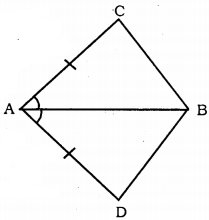To Prove: ∆ABC ≅ ∆ABD
Proof: In ∆ABC and ∆ABD
AC = AD (Data)
∠CAB = ∠DAB .
∠A bisected
AB is common.
Here Side, angle, side rule is there.
∴ ∆ABC ≅ ∆ABD.

Question 2.
ABCD is a quadrilateral in which AD = BC and ∠DAB = ∠CBA. Prove that
(1) ∆ABD ≅ ∆BAC
(2) BD = AC
(3) ∠ABD = ∠BAC A
Solution:
Data: ABCD is a quadrilateral.
AD = BC and ∠DAB = ∠CBA.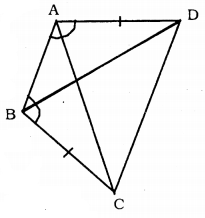To Prove:
(1) ∆ABD ≅ ∆BAC
(2) BD = AC
(3) ∠ABD = ∠BAC
Proof: (i) In ∆ABD and ∆BAC
AD = BC (Data)
∠DAB = ∠CBA (Data)
AB is common SAS postulate.
∴ ∆ABD ≅ ∆BAC.

(ii) ∆ABD ≅ ∆BAC.
∵ Corresponding sides are equal.
∴ BD = AC.

(iii) ∆ABD ≅ ∆BAC. (Proved)
Equal sides of Adjacent angles are equal.
As we have AD = BC,
The adjacent angle for AD is ABD
The adjacent angle for BC is BAC
∴∠ABD = ∠BAC.

Question 3.
AD and BC are equal perpendiculars to a line segment AB. Show that CD bisects AB.
Solution:
Data: AD and BC are equal perpendiculars to a line segment AB.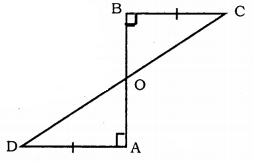To Prove: CD Bisects AB.
Proof: In ∆CBO and ∆DAO,
BC = AD
∠CBO = ∠DAO = 90°
∠BOC = ∠AOD (Vertically opposite angles)
SAS postulate,
∴ ∆CBO = ∆DAO ∴ OA = OB
∴ CD bisects AB at ‘O’.

Question 4.
l and m are two parallel lines intersected by another pair of parallel lines p and q. Show that ∆ABC ≅ ∆CDA.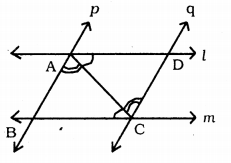Solution:
Data: l and m are two parallel lines intersected by another pair of parallel lines p and q.
To Prove: ∆ABC ≅ ∆CDA
Proof: In AABC and ACDA,
∠ACB = ∠DAC [∵ Alternate angles.]
∠BAC = ∠ACD
AC is common.
A.S.A. postulate.
∆ABC ≅ ∆CDA

Question 5.
Line 1 is the bisector of an angle ∠A and B is any point on l. BP and BQ are perpendiculars from B to the arms of ∠A. Show that :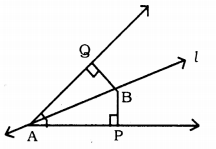(i) ∆APB ≅ ∆AQB
(ii) BP = BQ or B is equidistant from the arms of ∠A.
Solution:
Data: Line l is the bisector of an angle ∠A and B is any point on l.
BP and BQ are perpendiculars from B to the arms of ∠A.
To Prove:
(i) ∆APB ≅ ∆AQB
(ii) BP = BQ or B is equidistant from the arms of ∠A.
Proof: In ∆APB and ∆AQB
∠APB = ∠AQB = 90°
∠PAB = ∠QAB (∵ l bisects ∠A).
AB is common.
∴ ∆APB ≅ ∆AQB (ASA postulate)
∴ BP = BQ.
B is equidistant from the arms of ∠A.

Question 6.
In AC = AE, AB = AD and ∠BAD = ∠EAC. Show that BC = DE.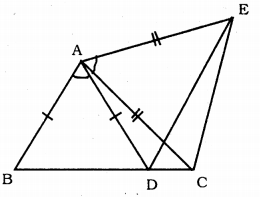Solution:
Data: AC = AE, AB = AD and ∠BAD = ∠EAC
To Prove : BC = DE
Proof: In ∆ABC and ∆EAD,
AB = AD (Data)
AC = AE (Data)
∆BAC = ∆EAD
(∵∠BAD + ∠DAC = AEAC + ∠DAC and ∠DAC = ∠DAC.)
Side – Angle – Side (SAS) Postulate
∴ ∆ABC = ∆EAD
∴ BC = DE.

Question 7.
AB is a line segment and P is its midpoint. D and E are points on the same side of AB such that ∠BAD = ∠ABE and ∠EPA = ∠DPB. Show that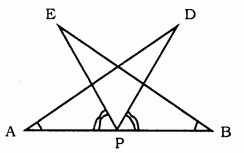(i) ∆DAP ≅ ∆EBP
(ii) AD = BE.
Solution:
Data: AB is a line segment and P is its mid-point. D and E are points on the same side of AB such that ∠BAD= ∠ABE and ∠EPA = ∠DPB.
To Prove :
(i) ∆DAP ≅ ∆EBP
(ii) AD = BE
Proof : (i) In ∆DAP and ∆EBP,
AP = BP (∵ P is the mid-point of AB)
∠BAD = ∠ABE (Data)
∠APD = ∠BPE
∵ (∠EPA = ∠DPB Adding ∠EPD to both sides)
∠EPA + ∠EPD = ∠DPB + ∠EPD
∴ ∠APD = -BPE.
Now, Angle, Side, Angle postulate.
∴ ∆DAP ≅ ∆EBP.

(ii) As it is ∆DAP ≅ ∆EBP,
Three sides and three angles are equal to each other.
∴ AD = BE.

Question 8.
In the right triangle ABC, right-angled at C, M is the mid-point of hypotenuse AB. C is joined to M and produced to a point D such that DM = CM. Point D is joined to point B. Show that :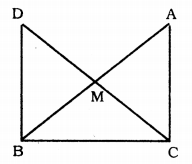(i) ∆AMC ≅ ∆BMD
(ii) ∠DBC is a right angle.
(iii) ∆DBC ≅ ∆ACB
(iv) CM = $$\frac{1}{2}$$ AB.
Solution:
Data: In a right triangle ABC, right-angled at C, M is the mid-point of hypotenuse AB.
C is joined to M and produced to a point D such that DM = CM.
Point D is joined to point B.
To Prove:
(i) ∆AMC ≅ ∆BMD
(ii) ∠DBC is a right angle.
(iii) ∆DBC ≅ ∆ACB
(iv) CM = $$\frac{1}{2}$$ AB.
Proof: (i) In ∆AMC and ∆BMD,
BM = AM (∵ M is the mid-point of AB)
DM = CM (Data)
∠BMD = ∠AMC (Vertically opposite angles)
Now, Side, Angle, Side postulate.
∴ ∆AMC ≅ ∆BMD

(ii) ∆AMC ≅ ∆BMD (Proved)
Adding AMBC to both sides,
∆BMD + ∆MBC = ∆AMC + ∆MBC
∆DBC ≅ ∆ACB.
∴ AB Hypotenuse = DC Hypotenuse
∠ACB = ∠DBC = 90°
∴ ∠DBC is a right angle.

(iii) In ∆DBC and ∆ACB,
DB = AC
∵ ∆DMB ≅ DMC (Proved)
∠DBC = ∠ACB (Proved)
BC is Common.
Side, angle, side postulate.
∴ ∠DBC ≅ ∆ACB.

(iv) ∆DBC ≅ ∆ACB (proved)
Hypotenuse AB = Hypotenuse DC
$$\frac{1}{2}$$ AB = $$\frac{1}{2}$$ DC
$$\frac{1}{2}$$ AB = CM
∴ CM = $$\frac{1}{2}$$AB.

We hope the KSEEB Solutions for Class 9 Maths Chapter 5 Triangles Ex 5.1 helps you. If you have any query regarding Karnataka Board Class 9 Maths Chapter 5 Triangles Exercise 5.1, drop a comment below and we will get back to you at the earliest.